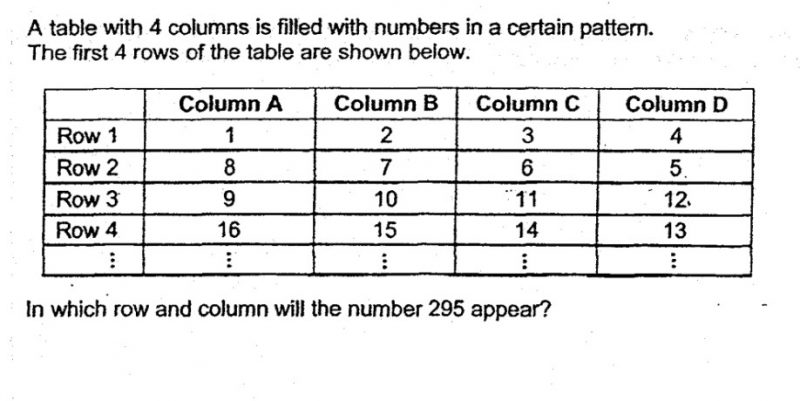# Questionhi! can help us with this qn? tq!

295/4 = 73.75 ——–> Row 74
295/8 = 36R7 ——-> Column B

Ans : Row 74 column B.

0 Replies 0 Likes

If the row number is odd, the numbers go in an ascending order, the number in Column D is the factor of 4.

If the row number is even, the numbers go in descending order, the number in Column A is the factor of 4.

We know that 292=4*73 appears in Row 73, Column D, so 293 is in Row 74, Column B.

0 Replies 0 Likes

When the numbers in the table are divided by 8, we get

 Column A Column B Column C Column D Row 1 0R1 0R2 0R3 0R4 Row 2 1R0 0R7 0R6 0R5 Row 3 1R1 1R2 1R3 1R4 Row 4 2R0 1R7 1R6 1R5 Row 5 2R1 2R2 2R3 2R4 Row 6 3R0 2R7 2R6 2R5

Observe that numbers with remainders 0 & 1 will always be in Column A, remainders 2 & 7 in Column B, remainders 3 & 6 in Column C, and remainders 4 & 5 in Column D.

Next, observe that for remainders 1,2,3,4, the corresponding row number is (quotient × 2) + 1

Row number for remainders 5,6,7 is (quotient × 2) + 2

Row number for remainder 0 is simply (quotient × 2)

So, when 295 is divided by 8, we get 36R7.

The row number is (36 × 2) + 2 = 74

Therefore, 295 will be in Row 74 Column B